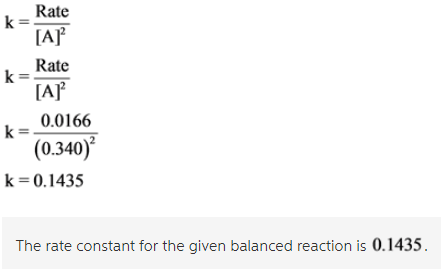# Using the given data, determine the rate constant of this reaction. A+2B ? > C+D

Using the given data, determine the rate constant of this reaction.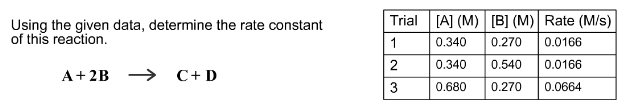Concepts and reason
Rate of a reaction is defined as change in the concentration of reactants and products per unit time. It is the decrease in the concentration of reactants and the increase in the concentration of products.

Fundamentals
Rate of a reaction is expressed in terms of rate law. Rate law expresses the relationship between the rate of the reaction and the concentration of reactants. The rate of a reaction is directly proportional to the concentration of reactants. Order of a reaction is the sum of the powers of the concentrations of the reactants that appears in the rate law.

Consider the first and second trials. The concentration of A is unchanged. The rate of the reaction stays same on doubling the concentration of B. The rate of reaction is first order with respect to B.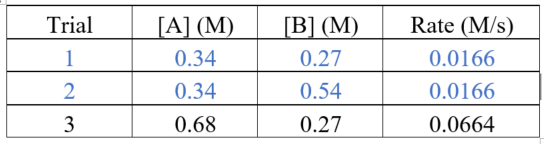Consider the first and third trials. The concentration of B is unchanged. The rate of the reaction has become four times on doubling the concentration of A.
The rate of reaction is second order with respect to B.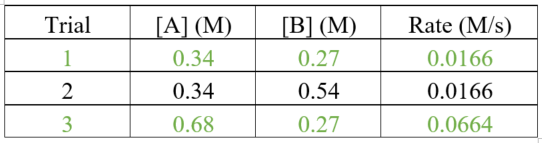Use the data from the first and second trials to calculate the value of rate constant.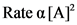Remove the proportionality sign by adding the proportionality constant, k.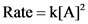On rearranging,